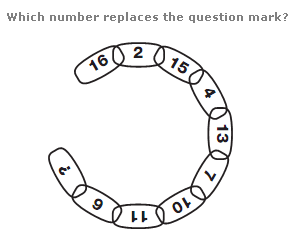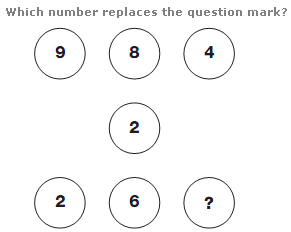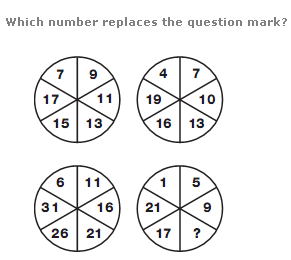# Puzzles - Number puzzles

### Exercise :: Number puzzlesAnswer : 16 Explanation : Moving clockwise, around alternate segments in the chain, one sequence decreases by 1, 2, 3 and 4 each time, while the other increases by 2, 3, 4 and 5.Answer : 7 Explanation : Starting with the numbers in the top row, and following straight lines through the centre of the diagram, subtract the middle number from the top number to give the corresponding value on the bottom row.Answer : 13 Explanation : In each circle, starting at the top left segment, numbers increase, as you move clockwise, by 2 for the upper left circle, 3 for the upper right, 4 for the lower right and 5 for the lower left.